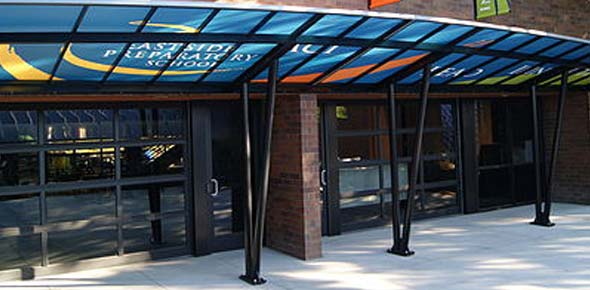# MCAS Prep Questions

10 Questions | Attempts: 1012
ShareSettingsQuestions reviewing sample questions on the MA MCAS test.

• 1.
If a solid object is taken from Earth far into space, which of the following measurements of the object will change MOST?
• A.

Density

• B.

Mass

• C.

Volume

• D.

Weight

• 2.
Which of the following is an example of a container that is filled with a pure substance rather than a mixture?
• A.

A tire filled with air

• B.

A jar filled with salt water

• C.

A balloon filled with helium

• D.

A glass filled with chocolate milk

• 3.
The table below shows the number of each kind of atom that makes up one molecule of the compounds sucrose and ethanol. Compound: sucrose (12 atoms of carbon, 11 atoms of oxygen, and 22 atoms of hydrogen)  and: ethanol (2 atoms of carbon, 1 atom of oxygen, and 6 atoms of hydrogen).Which of the following is the SAME for both compounds?
• A.

The mass of each molecule

• B.

The length of each molecule

• C.

The number of atoms in each molecule

• D.

The types of atoms in each molecule

• 4.
A student is given a sample of an unknown liquid to test in the laboratory. The student thinks that the liquid is water. Which of the following physical properties of the sample is MOST helpful to determine if the liquid is water?
• A.

Boiling point of the liquid

• B.

Color of the liquid

• C.

Volume of the liquid

• D.

Mass of the liquid

• 5.
Which of the following is formed when two elements combine chemically?
• A.

An atom

• B.

A compound

• C.

An electron

• D.

A mixture

• 6.
Mrs. Collette measured the mass of a closed beaker containing several ice cubes. The mass was 100 g. An hour later, after the ice cubes had melted, Mrs. Collette measured the mass of the beaker again. What was the mass of the beaker of water the second time?
• A.

50 g

• B.

100 g

• C.

75 g

• D.

125 g

• 7.
New carpet is being measured for a room that a 5.5 m by 4.6 m. On a measuring tape, what is the smallest unit that is NECESSARY to measure the room accurately for the carpet?
• A.

Millimeters

• B.

Kilometers

• C.

Meters

• D.

Centimeters

• 8.
Sugar is composed of carbon, hydrogen, and oxygen. Sugar is an example of which of the following?
• A.

An atom

• B.

An electron

• C.

A compound

• D.

A mixture

• 9.
The surface of a heated metal object measures 120 degrees celsius. It is dropped into a bucket filled with water measuring 10 degrees celsius. Which of the following is most likely to occur?
• A.

Both the water and metal will adjust to the same temperature below 10 degrees celsius.

• B.

The water and metal will adjust to different temperatures above 120 degrees celsius.

• C.

The water will remain the same temperature, but the metal will cool to 10 degrees celsius.

• D.

The water and metal will adjust to the same temperature between 10 and 120 degrees celsius.

• 10.
A pot of water was heated on a stove until the water boiled. Which of the following BEST explains why the water was able to boil?
• A.

The cold water absorbed heat from the pot.

• B.

The hot stove absorbed cold from the pot.

• C.

The hot stove gave off heat to the surrounding air.

• D.

The cold water gave off cold to the surrounding air.

## Related TopicsBack to top
×

Wait!
Here's an interesting quiz for you.